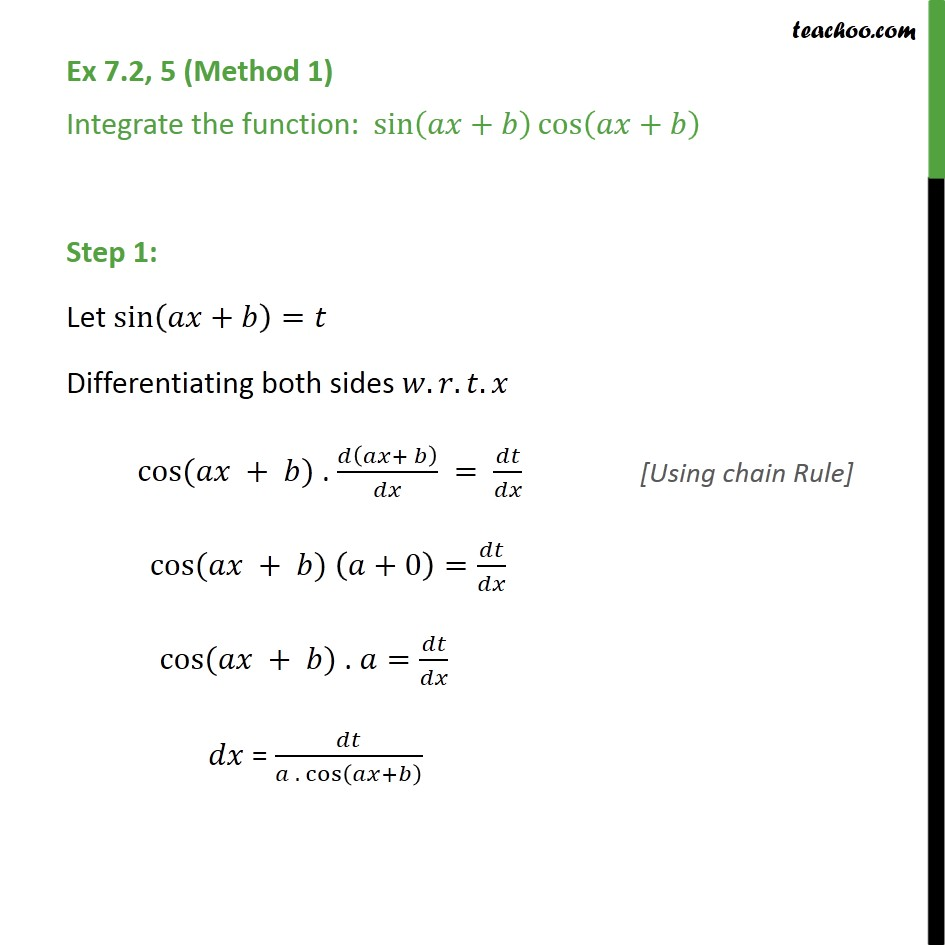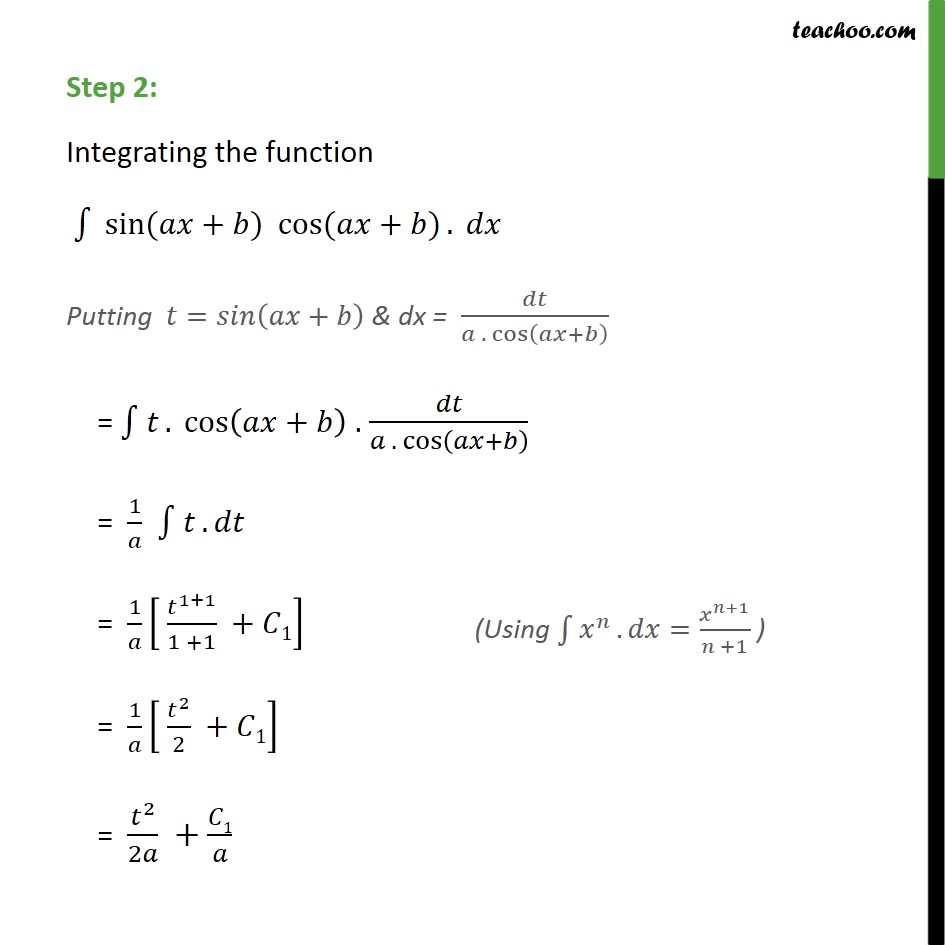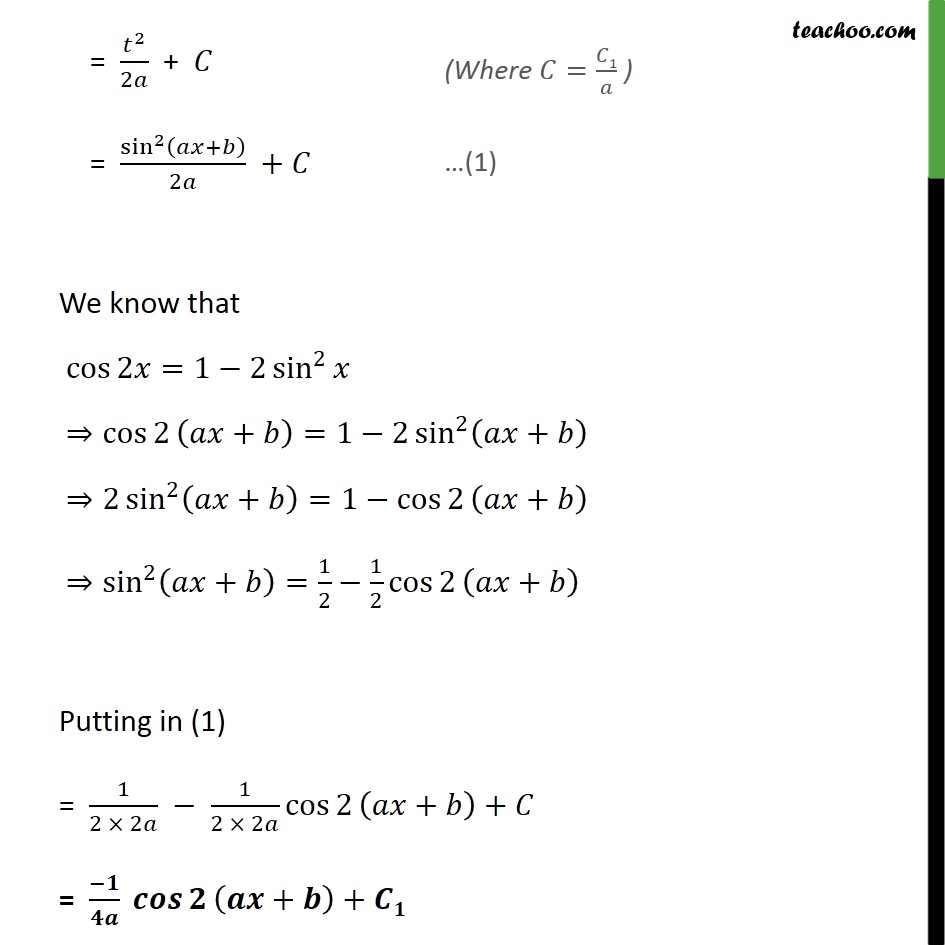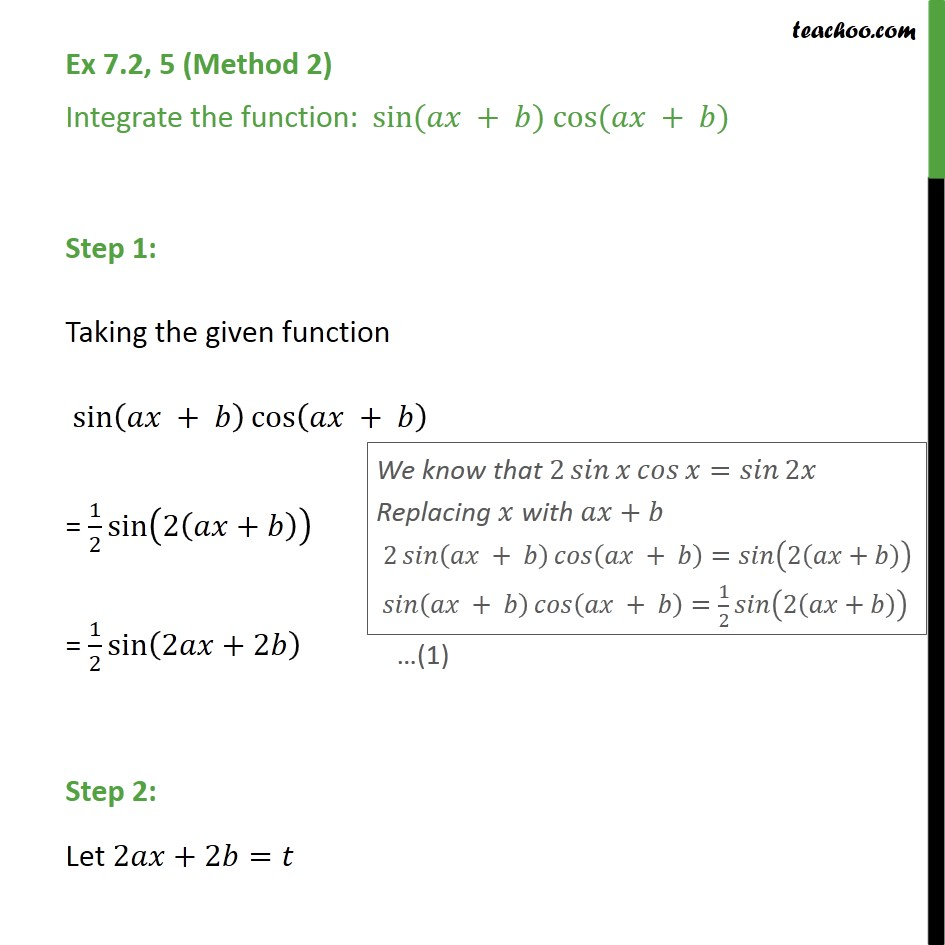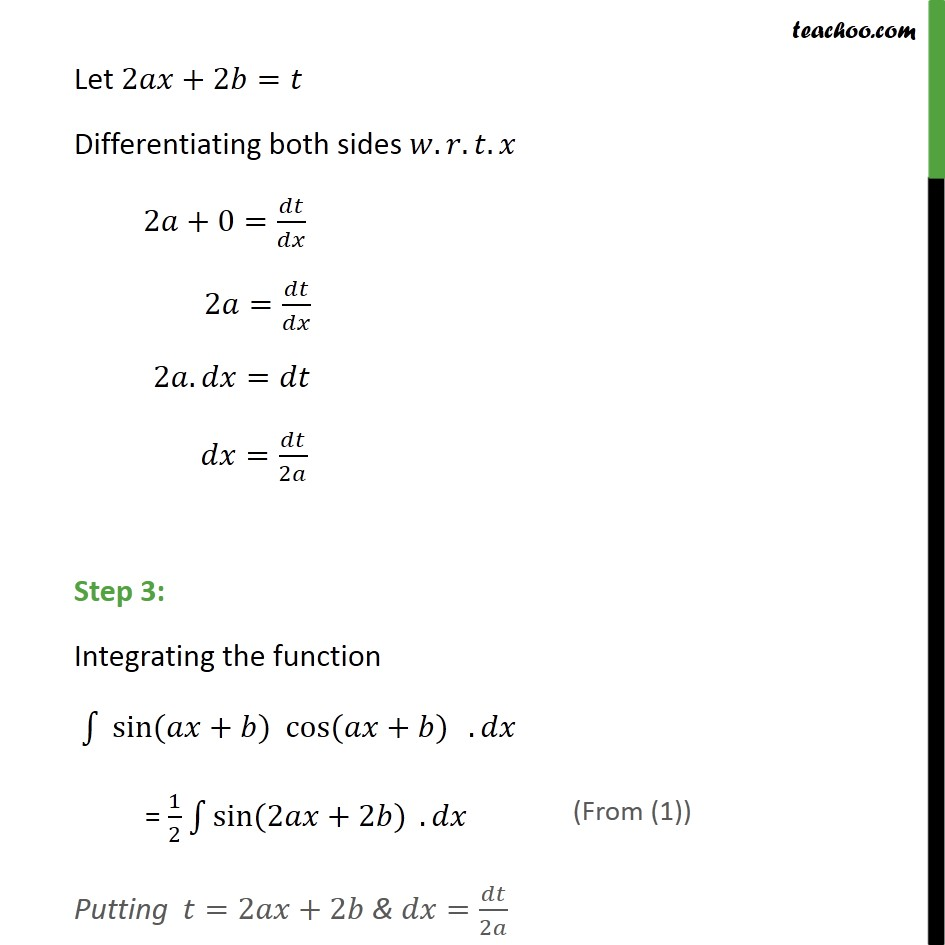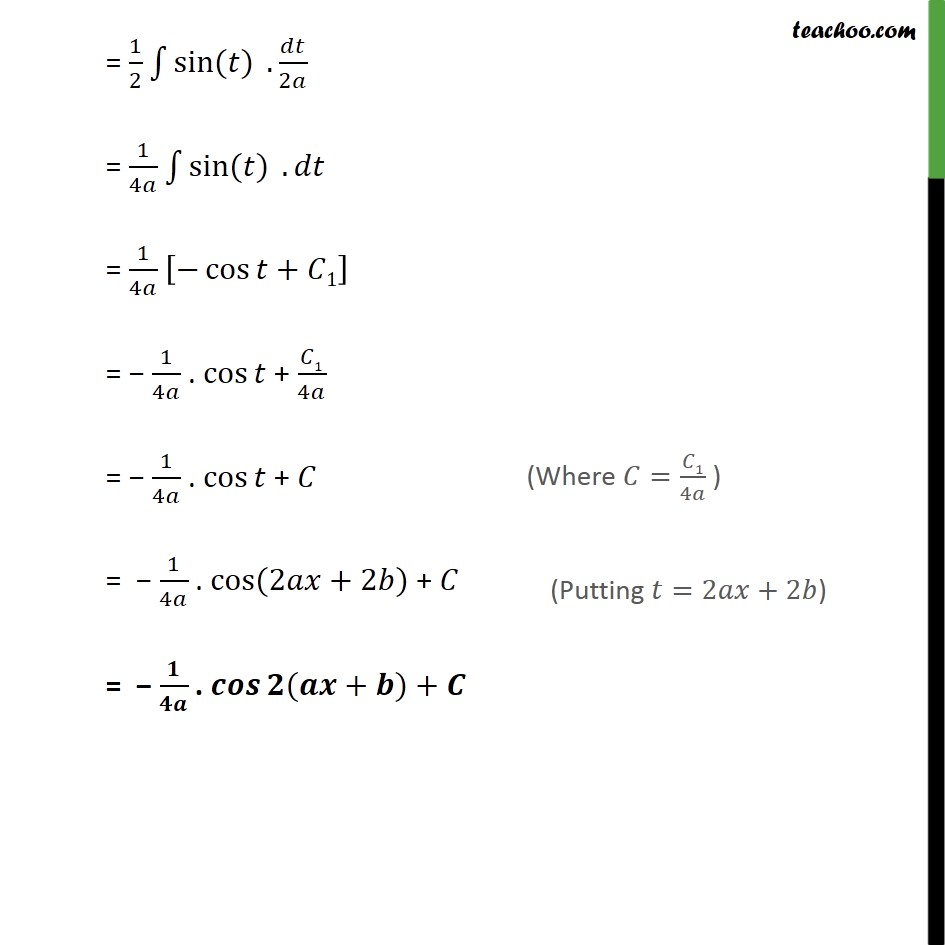1. Chapter 7 Class 12 Integrals
2. Serial order wise
3. Ex 7.2

Transcript

Ex 7.2, 5 (Method 1) Integrate the function: sin + cos + Step 1: Let sin + = Differentiating both sides . . . cos ( + ) . + = cos ( + ) +0 = cos ( + ) . = = . cos + Step 2: Integrating the function sin ( + ) cos ( + ) . Putting = + & dx = . cos + = . cos + . . cos + = 1 . = 1 1+1 1 +1 + 1 = 1 2 2 + 1 = 2 2 + 1 = 2 2 + = sin 2 + 2 + We know that cos 2 =1 2 sin 2 cos 2 + =1 2 sin 2 + 2 sin 2 + =1 cos 2 + sin 2 + = 1 2 1 2 cos 2 + Putting in (1) = 1 2 2 1 2 2 cos 2 + + = + + Ex 7.2, 5 (Method 2) Integrate the function: sin ( + ) cos ( + ) Step 1: Taking the given function sin + cos + = 1 2 sin 2 + = 1 2 sin 2 +2 Step 2: Let 2 +2 = Let 2 +2 = Differentiating both sides . . . 2 +0= 2 = 2 . = = 2 Step 3: Integrating the function sin ( + ) cos ( + ) . = 1 2 sin (2 +2 ) . Putting =2 +2 & = 2 = 1 2 sin ( ) . 2 = 1 4 sin ( ) . = 1 4 cos + 1 = 1 4 . cos + 1 4 = 1 4 . cos + = 1 4 . cos (2 +2 ) + = . ( + ) +

Ex 7.2

Chapter 7 Class 12 Integrals
Serial order wise

About the AuthorDavneet Singh
Davneet Singh is a graduate from Indian Institute of Technology, Kanpur. He has been teaching from the past 10 years. He provides courses for Maths and Science at Teachoo.